Next: Pseudo GF. Rectangular coordinate, Up: Rectangular Coordinates. Finite Bodies, Previous: Rectangle, steady GF, single-

### Parallelepiped, steady GF, double- and triple- sum forms.

The steady GF for a rectangular parallelepiped satisfies the following equations: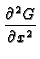+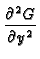+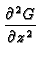= -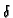(x - x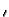)(y - y)(z - z) (12) 0 < x < L;  0 < y < W;  0 < z < H ki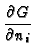+ hiG = 0 for faces i = 1, 2,..., 6 (13)

The units of the 3D GF are [meters-1]. The 3D GF may be stated in one of four alternative forms, discussed below.

Triple-Summation Form for the 3D Parallelepiped
The GF may be found by separation of variables in all three coordinates in the form
 G3D(x, y, z | x, y, z) =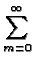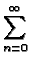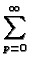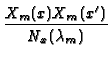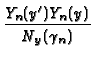x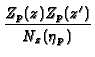x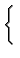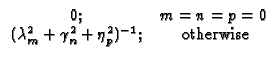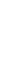Here Nx(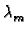), Ny(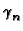) and Nz(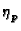) denote the norms of the mth x-direction eigenfunction, the nth y-direction eigenfunction, and the pth z-direction eigenfunction, respectively. Eigenfunctions Xm(x) and norm Nx() are given in Tables 2 and 3; related y -and z -axis quantities can be found from these tables by substituting appropriate values; for example in the z-direction substitute z, H, andin place of x, L, and. The triple summation GF also arises naturally from transient GF which are integrated over time. The triple sum form of the 3D GF generally has poor convergence properties; the double-summation GF discussed next is recommended for numerical computation.

Double-Summation Form for the 3D Parallelepiped
The GF stated below contains a double summation that involves two eigenfunctions, their norms, and a kernel function P, as follows:

 G3D(x, y, z | x, y, z) =Pnp(x, x) (14)

Kernel functions Pnp are identical to the functions already introduced in equation (14) except that for the 3D case, replace eigenvalueby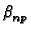defined by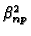=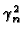+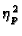. That the above double-summation form satisfies equation (15) can be demonstrated by direct substitution; however the identity for the Dirac delta function, equation (7), is needed for both(y - y) and for(z - z). The n = 0 and p = 0 terms are only needed when Y22Z22 is part of the GF number; in this special case the= 0 kernel function is needed; it is P0, described earlier for the 2D and 1D GF.

Alternative Double-summation Forms for the Parallelepiped
In the GF given above the kernel functions Pnp are placed along the x-axis. For a given parallelepiped there are three different double-sum series expansions for the GF, depending on whether the kernel functions are placed along the x-, y-, or z-axis. Each of the alternative forms of the GF, although a different series expansion, represent the same unique solution. The convergence behavior of alternative forms of the 3D GF are generally complementary. Where one converges slowly, another may generally be found that converges rapidly.Next: Pseudo GF. Rectangular coordinate, Up: Rectangular Coordinates. Finite Bodies, Previous: Rectangle, steady GF, single-
Kevin D. Cole
2002-12-31